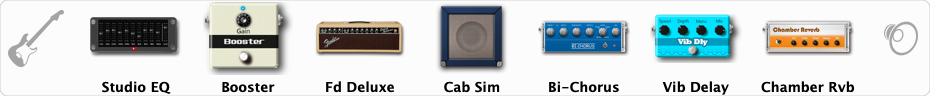# Mac Demarco 2012-2015 tone

Discussion in 'ToneLib-GFX presets' started by thetwillz, Oct 6, 2019.

1. Mac Demarco 2012-2015 tone

Preset name: Mac Demarco 2012-2015 tone

Effects chain:Effect: "Studio EQ" (Dynamics / Filter), active - "yes"
"31 Hz" = 0
"62 Hz" = 0
"125 Hz" = 0
"250 Hz" = 0
"500 Hz" = 0
"1 kHz" = 0
"2 kHz" = 0
"4 kHz" = 0
"8 kHz" = -1
"16 kHz" = -3
"above 16 kHz" = 0
"Level (dB)" = 0

Effect: "Booster" (Dynamics / Filter), active - "yes"
"Gain" = 29

Effect: "Fd Deluxe" (Amp simulators), active - "yes"
"Gain" = 50
"Bass" = 51
"Middle" = 62
"Treble" = 71
"Presence" = 47
"Master" = 50
"Output" = 50
"Level (dB)" = 0

Effect: "Cab Sim" (Cabinets), active - "yes"
"Model" = 2x10" Vibrolux
"Level (dB)" = 0

Effect: "Bi-Chorus" (Modulation / Sfx), active - "yes"
"Speed A" = 3.2
"Speed B" = 4.0
"Depth" = 40
"Reso" = 0
"Mode" = OppPhase
"Mix" = 100

Effect: "Vib Delay" (Modulation / Sfx), active - "yes"
"Speed" = 5.1
"Depth" = 42
"Feedback" = 64
"Time" = 5.7
"Mix" = 27
"Mode" = 1

Effect: "Chamber Rvb" (Reverb), active - "yes"
"Time" = 6.1
"PreDelay" = 23
"LoDamp" = 24
"HiDamp" = 33
"Mix" = 43

Note: You will need to download and install the ToneLib-GFX software to use the preset.

File size:
644 bytes
Views:
8,723